AVL Tree

# AVL Tree

#### In this tutorial, you will learn what an avl tree is. Also, you will find working examples of various operations performed on an avl tree in C, C++, Java and Python.

AVL tree is a self-balancing binary search tree in which each node maintains an extra information called as balance factor whose value is either -1, 0 or +1.

AVL tree got its name after its inventor Georgy Adelson-Velsky and Landis.

## Balance Factor

Balance factor of a node in an AVL tree is the difference between the height of the left subtree and that of right subtree of that node.

Balance Factor = (Height of Left Subtree - Height of Right Subtree) or (Height of Right Subtree - Height of Left Subtree)

The self balancing property of an avl tree is maintained by the balance factor. The value of balance factor should always be -1, 0 or +1.

An example of a balanced avl tree is: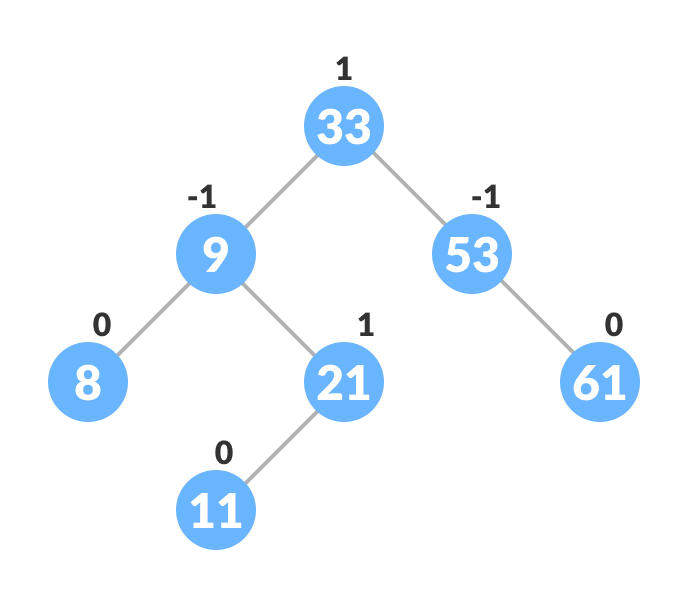## Operations on an AVL tree

Various operations that can be performed on an AVL tree are:

## Rotating the subtrees in an AVL Tree

In rotation operation, the positions of the nodes of a subtree are interchanged.

There are two types of rotations:

### Left Rotate

In left-rotation, the arrangement of the nodes on the right is transformed into the arrangements on the left node.

Algorithm

1. Let the initial tree be: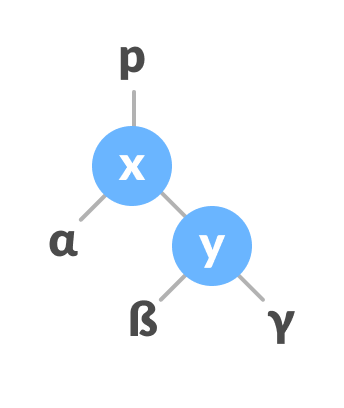2. If y has a left subtree, assign x as the parent of the left subtree of y.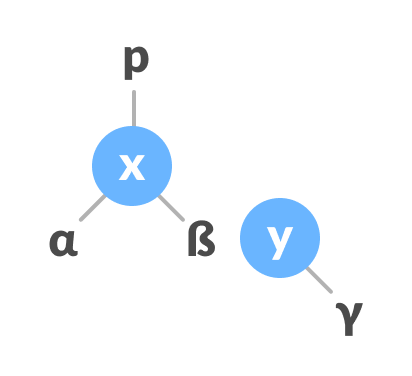3. If the parent of x `p` is `NULL`, make y as the root of the tree.
4. Else if x is the left child of p, make y as the left child of p.
5. Else assign y as the right child of p.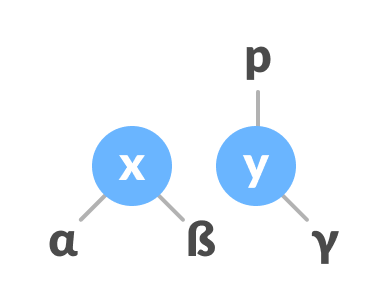6. Make y as the parent of x.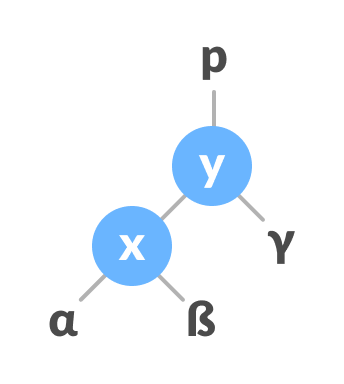### Right Rotate

In left-rotation, the arrangement of the nodes on the left is transformed into the arrangements on the right node.

1. Let the initial tree be: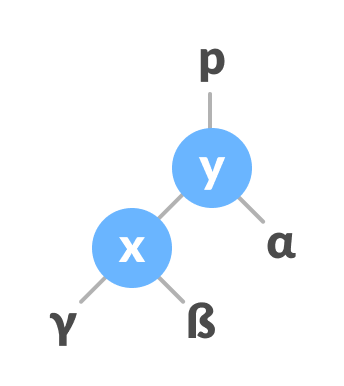2. If x has a right subtree, assign y as the parent of the right subtree of x.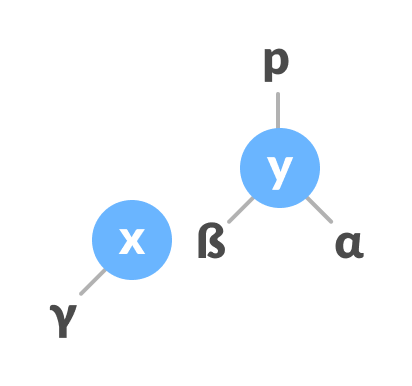3. If the parent of y is `NULL`, make x as the root of the tree.
4. Else if y is the right child of its parent p, make x as the right child of p.
5. Else assign x as the left child of p.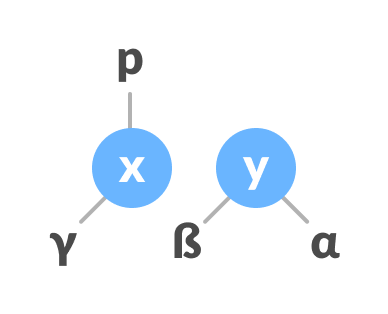6. Make x as the parent of y.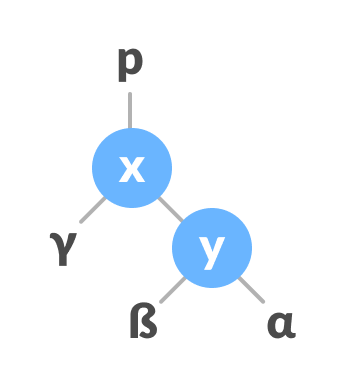### Left-Right and Right-Left Rotate

In left-right rotation, the arrangements are first shifted to the left and then to the right.

1. Do left rotation on x-y.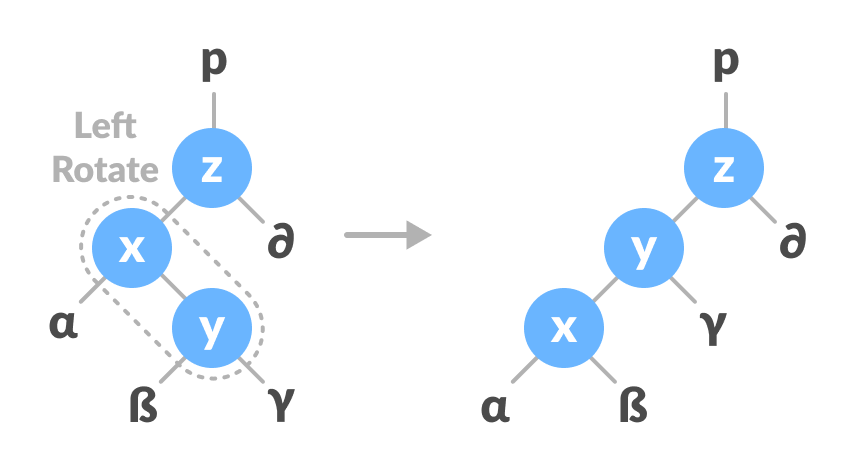2. Do right rotation on y-z.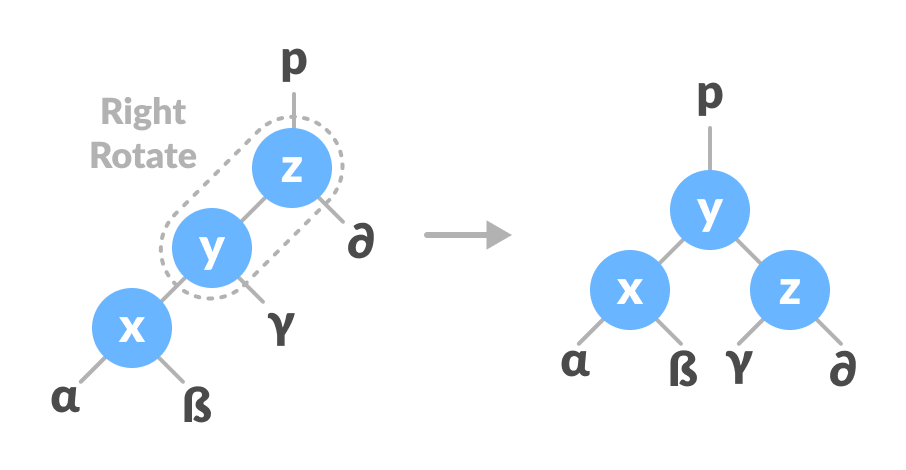In left-right rotation, the arrangements are first shifted to the right and then to the left.

1. Do right rotation on x-y.2. Do left rotation on z-y.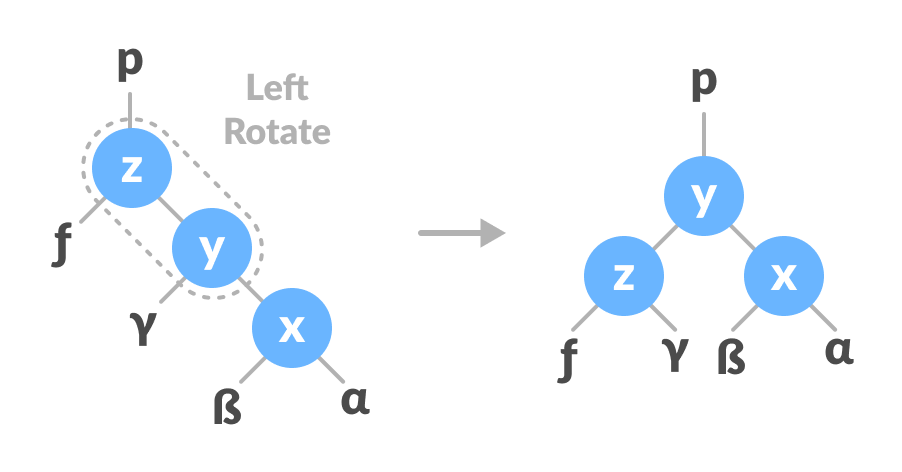## Algorithm to insert a newNode

A newNode is always inserted as a leaf node with balance factor equal to 0.

1. Let the initial tree be: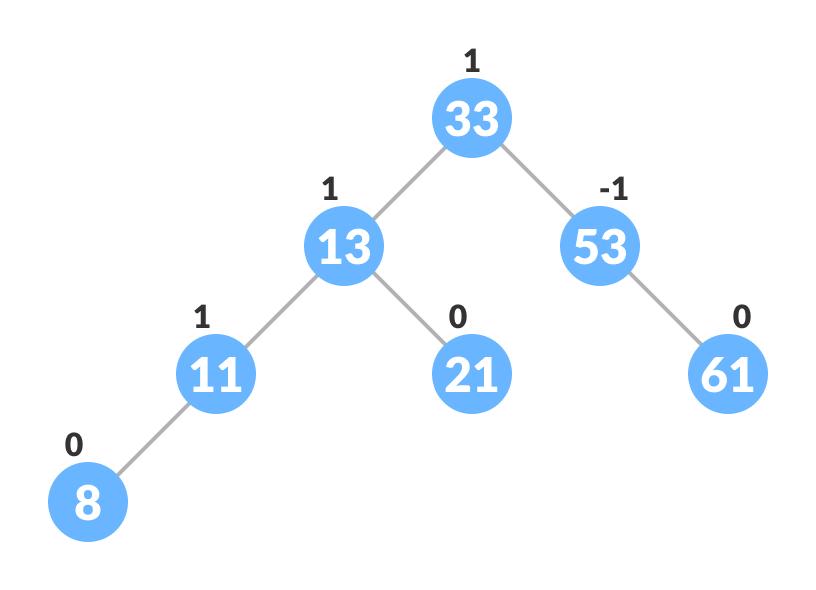Let the node to be inserted be:2. Go to the appropriate leaf node to insert a newNode using the following recursive steps. Compare newKey with rootKey of current tree.
1. If newKey < rootKey, call insertion algorithm on left subtree of current node until the leaf node is reached.
2. Else if newKey > rootKey, call insertion algorithm on the right subtree of current node until the leaf node is reached.
3. Else, return leafNode.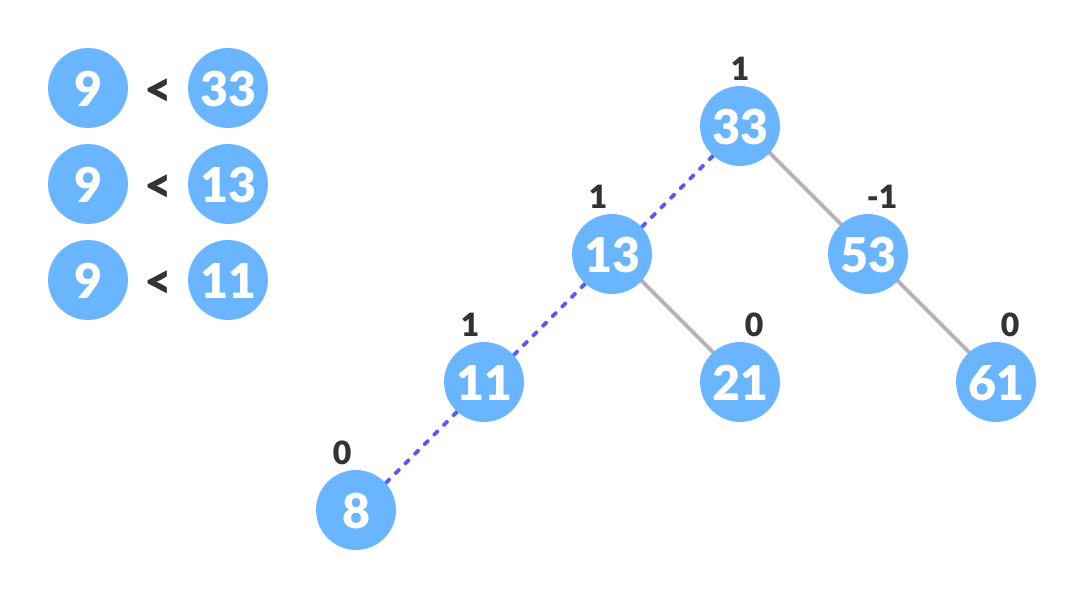3. Compare leafKey obtained from above steps with newKey:
1. If newKey < leafKey, make newNode as the leftChild of leafNode.
2. Else, make newNode as rightChild of leafNode.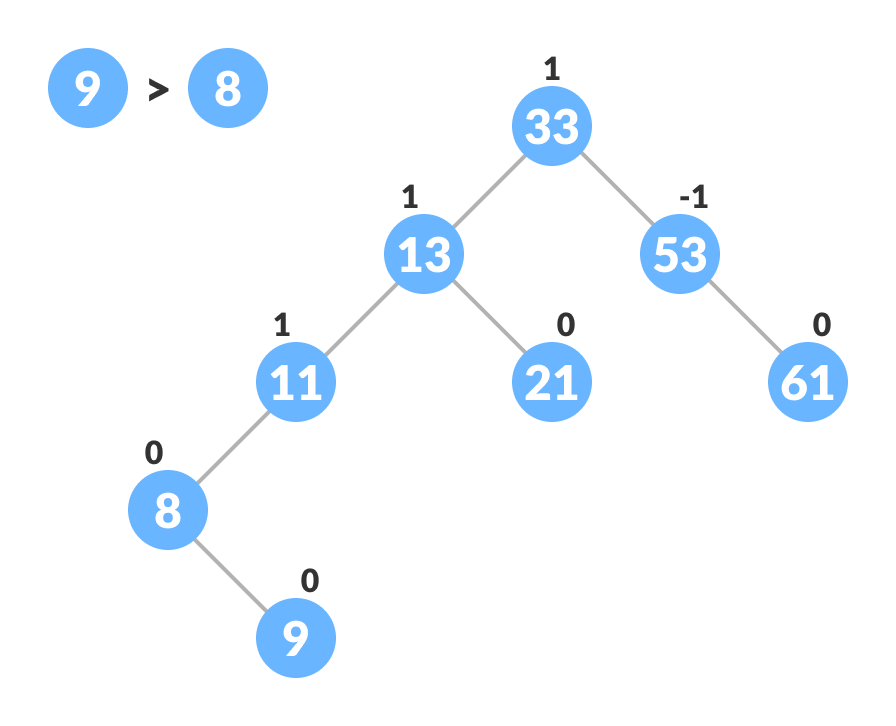4. Update balanceFactor of the nodes.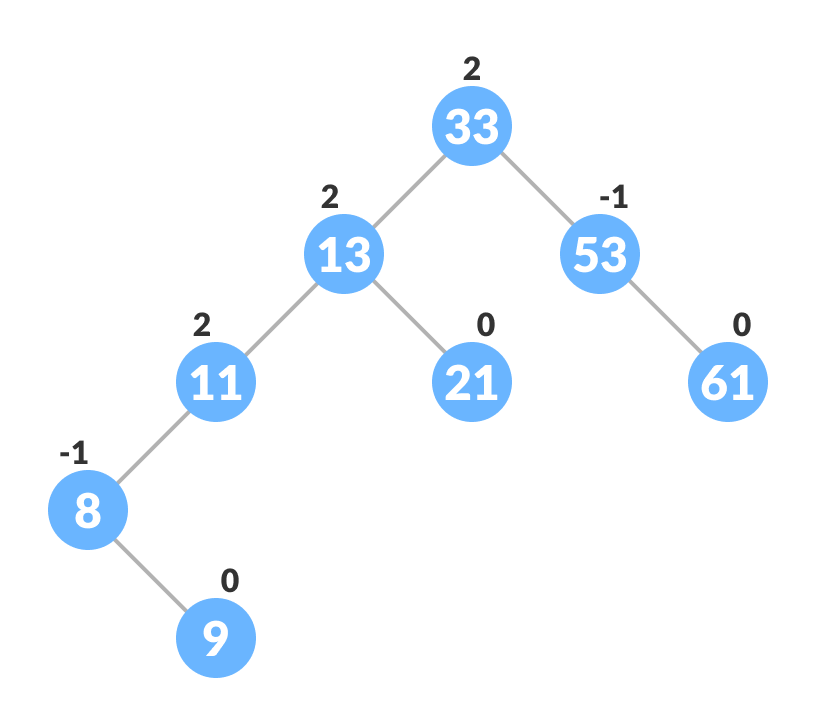5. If the nodes are unbalanced, then rebalance the node.
1. If balanceFactor > 1, it means the height of the left subtree is greater than that of the right subtree. So, do right rotation or left-right rotation
1. If newNodeKey < leftChildKey do right rotation.
2. Else, do left-right rotation.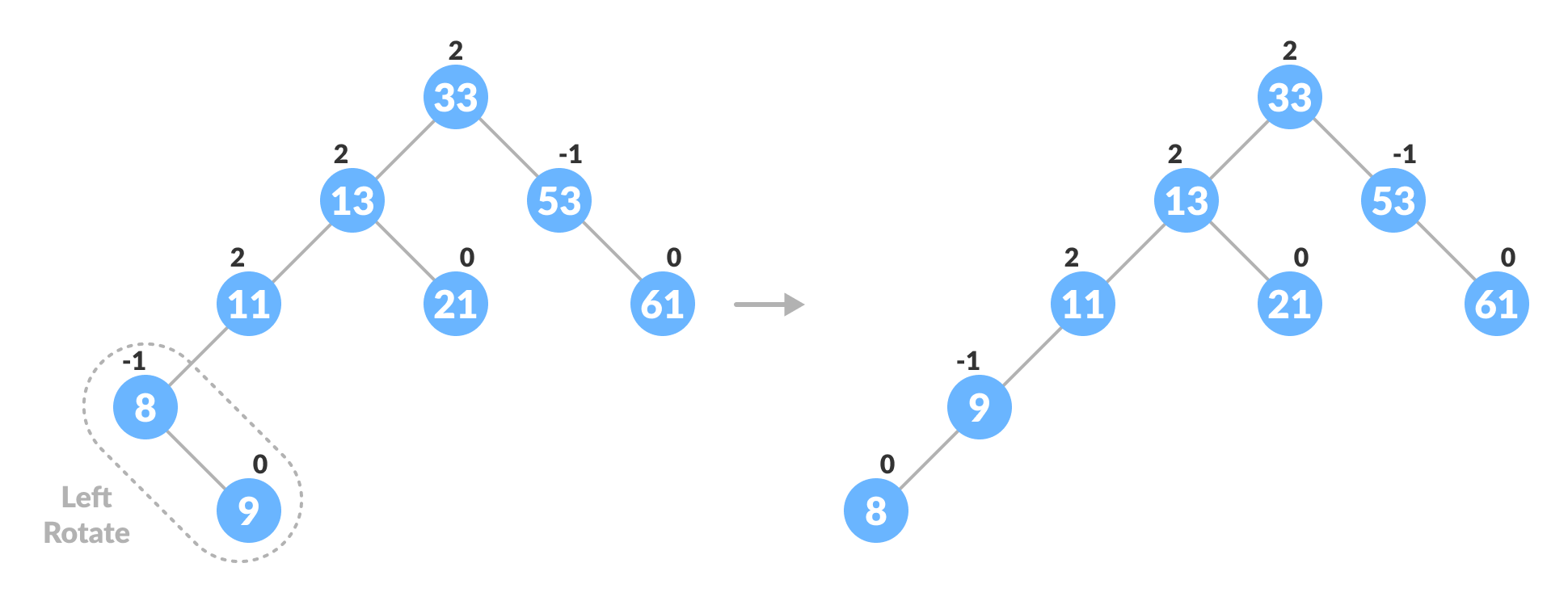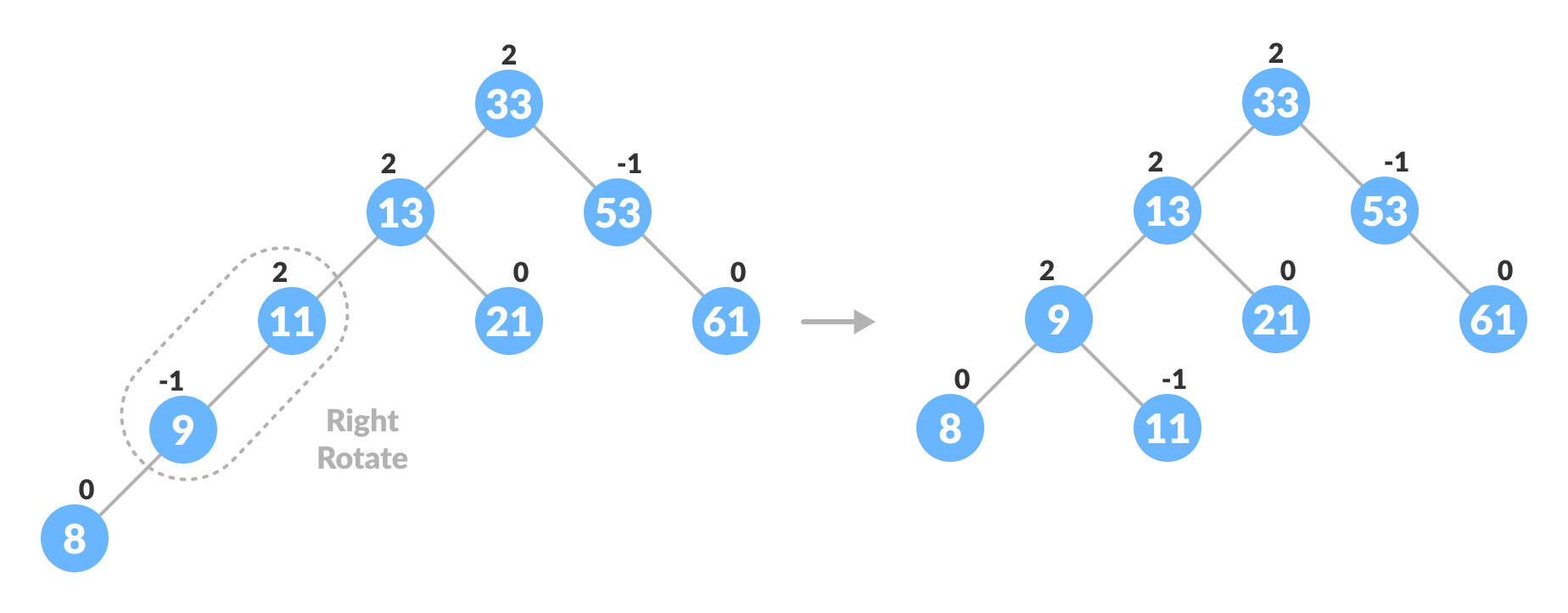2. If balanceFactor < -1, it means the height of the right subtree is greater than that of the left subtree. So, do right rotation or right-left rotation
1. If newNodeKey > rightChildKey do left rotation.
2. Else, do right-left rotation
6. The final tree is: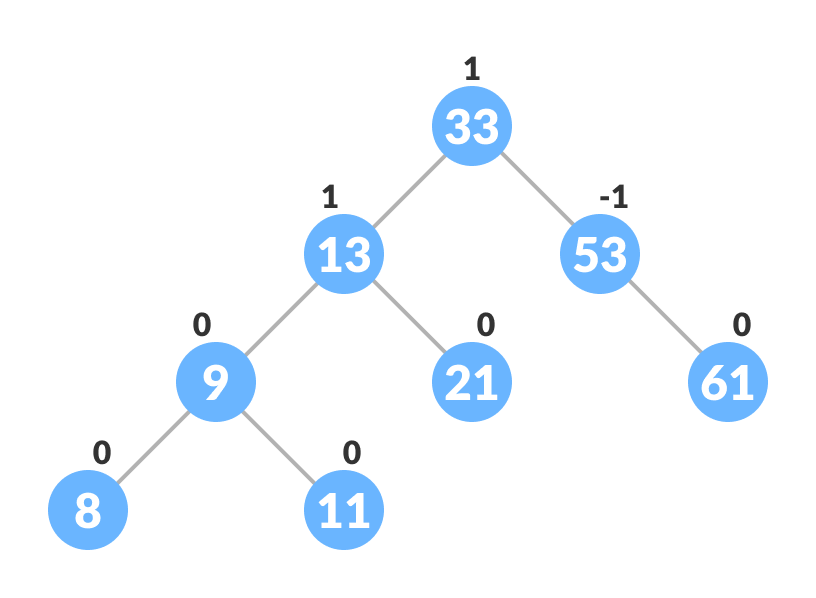## Algorithm to Delete a node

A node is always deleted as a leaf node. After deleting a node, the balance factors of the nodes get changed. In order to rebalance the balance factor, suitable rotations are performed.

1. Locate nodeToBeDeleted (recursion is used to find nodeToBeDeleted in the code used below).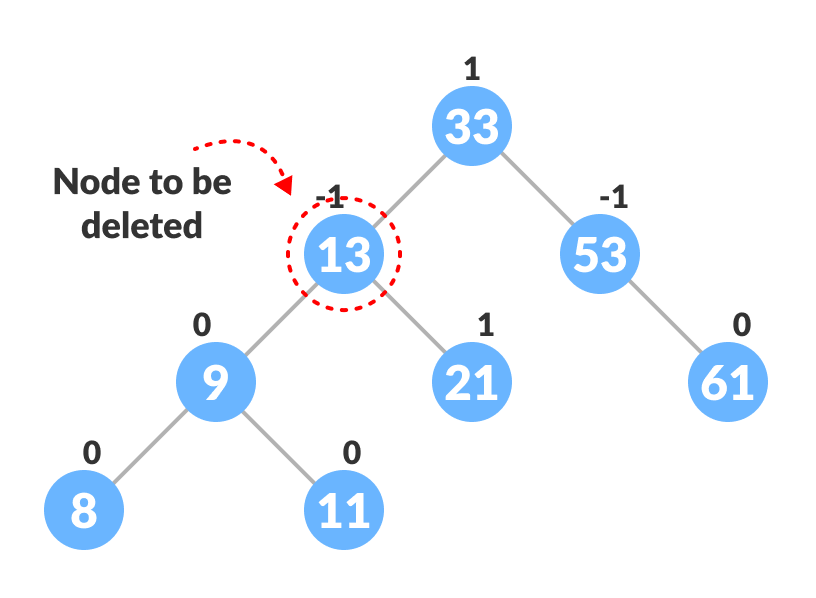2. There are three cases for deleting a node:
1. If nodeToBeDeleted is the leaf node (ie. does not have any child), then remove nodeToBeDeleted.
2. If nodeToBeDeleted has one child, then substitute the contents of nodeToBeDeleted with that of child. Remove the child.
3. If nodeToBeDeleted has two children, find the inorder successor w of nodeToBeDeleted (ie. node with minimum value of key in the right subtree).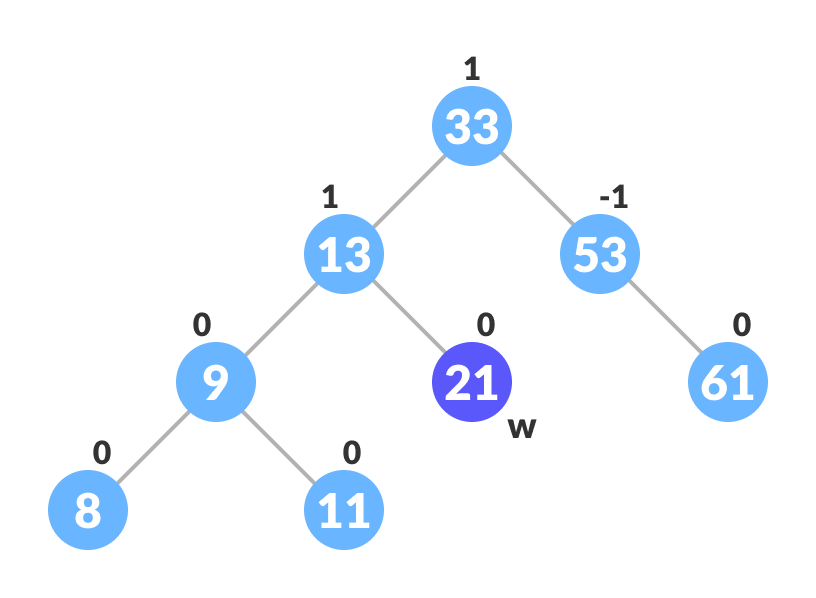1. Substitute the contents of nodeToBeDeleted with that of w.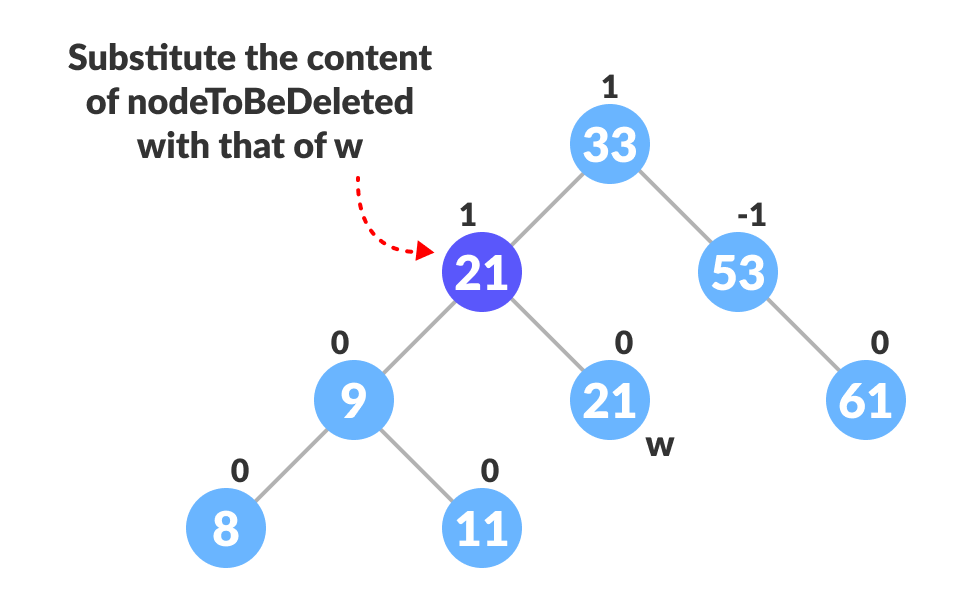2. Remove the leaf node w.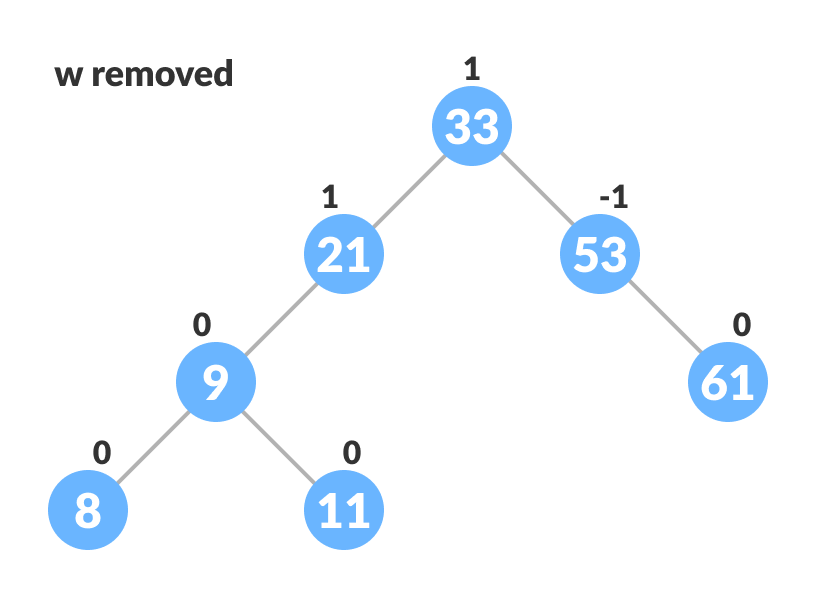3. Update balanceFactor of the nodes.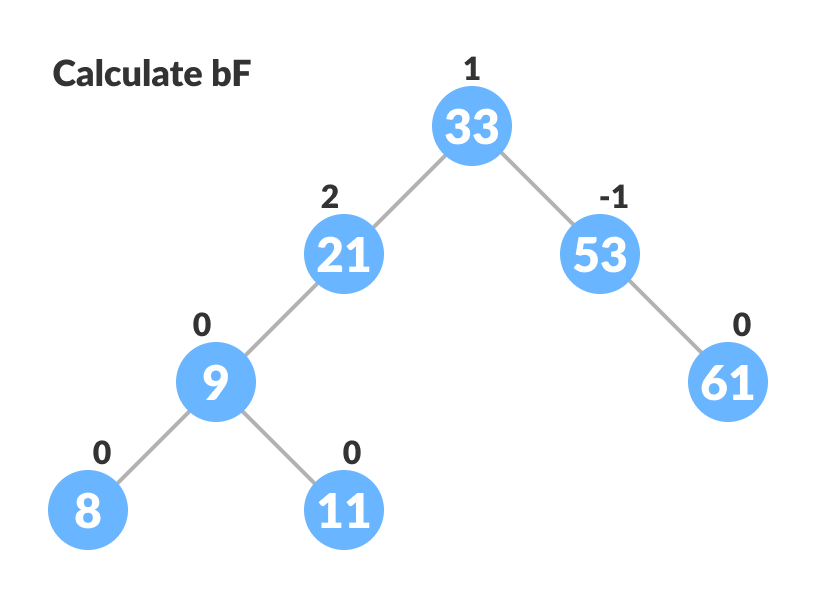4. Rebalance the tree if balance factor of any of the nodes is not equal to -1, 0 or 1.
1. If balanceFactor of currentNode > 1,
1. If balanceFactor of leftChild >= 0, do right rotation.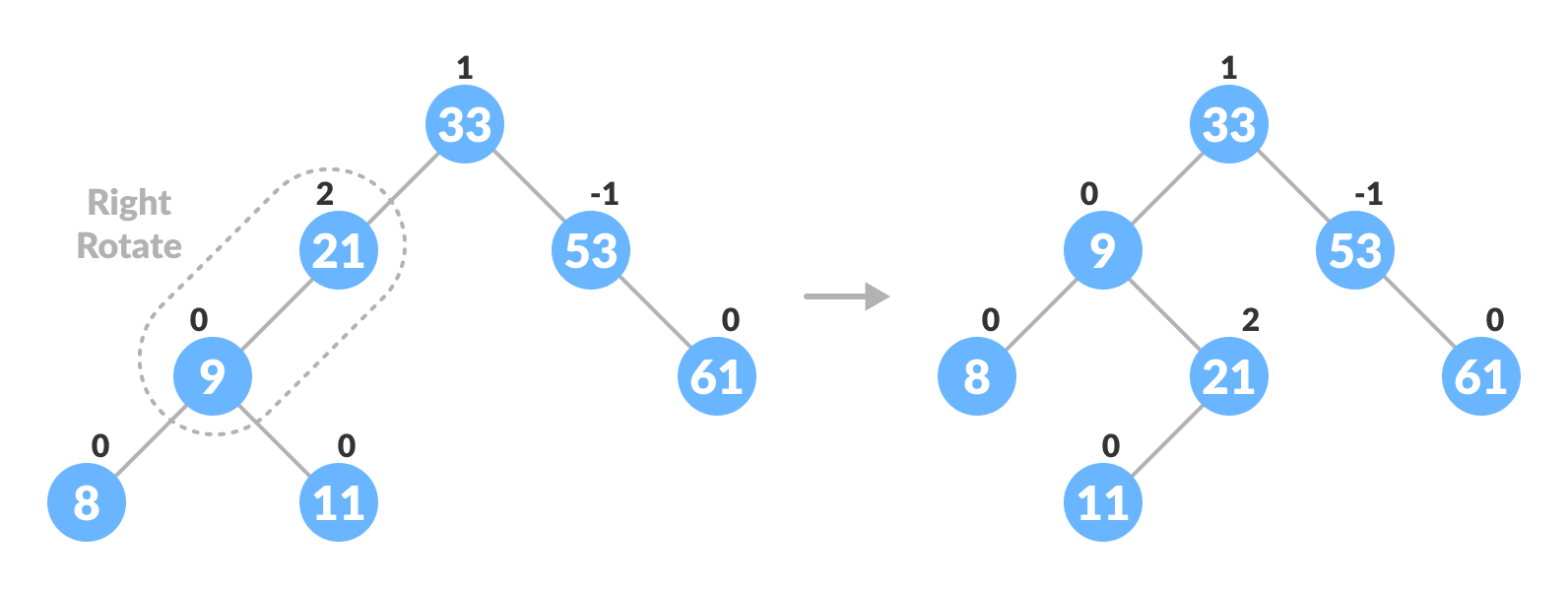2. Else do left-right rotation.
2. If balanceFactor of currentNode < -1,
1. If balanceFactor of rightChild <= 0, do left rotation.
2. Else do right-left rotation.
5. The final tree is:## Python, Java and C/C++ Examples

``````# AVL tree implementation in Python

import sys

class TreeNode(object):
def __init__(self, key):
self.key = key
self.left = None
self.right = None
self.height = 1

class AVL_Tree(object):

def insertNode(self, root, key):

if not root:
return TreeNode(key)
elif key < root.key:
root.left = self.insertNode(root.left, key)
else:
root.right = self.insertNode(root.right, key)

root.height = 1 + max(self.getHeight(root.left),
self.getHeight(root.right))

balanceFactor = self.getBalance(root)

if balanceFactor > 1:
if key < root.left.key:
return self.rightRotate(root)
else:
root.left = self.leftRotate(root.left)
return self.rightRotate(root)

if balanceFactor < -1:
if key > root.right.key:
return self.leftRotate(root)
else:
root.right = self.rightRotate(root.right)
return self.leftRotate(root)

return root

def delete(self, root, key):

if not root:
return root

elif key < root.key:
root.left = self.delete(root.left, key)

elif key > root.key:
root.right = self.delete(root.right, key)

else:
if root.left is None:
temp = root.right
root = None
return temp

elif root.right is None:
temp = root.left
root = None
return temp

temp = self.getMinValueNode(root.right)
root.key = temp.key
root.right = self.delete(root.right,
temp.key)

if root is None:
return root

root.height = 1 + max(self.getHeight(root.left),
self.getHeight(root.right))

balanceFactor = self.getBalance(root)

if balanceFactor > 1:
if self.getBalance(root.left) >= 0:
return self.rightRotate(root)
else:
root.left = self.leftRotate(root.left)
return self.rightRotate(root)

if balanceFactor < -1:
if self.getBalance(root.right) <= 0:
return self.leftRotate(root)
else:
root.right = self.rightRotate(root.right)
return self.leftRotate(root)

return root

def leftRotate(self, z):

y = z.right
T2 = y.left

y.left = z
z.right = T2

z.height = 1 + max(self.getHeight(z.left),
self.getHeight(z.right))
y.height = 1 + max(self.getHeight(y.left),
self.getHeight(y.right))

return y

def rightRotate(self, z):

y = z.left
T3 = y.right

y.right = z
z.left = T3

z.height = 1 + max(self.getHeight(z.left),
self.getHeight(z.right))
y.height = 1 + max(self.getHeight(y.left),
self.getHeight(y.right))

return y

def getHeight(self, root):
if not root:
return 0

return root.height

def getBalance(self, root):
if not root:
return 0

return self.getHeight(root.left) - self.getHeight(root.right)

def getMinValueNode(self, root):
if root is None or root.left is None:
return root

return self.getMinValueNode(root.left)

def preOrder(self, root):

if not root:
return

print("{0} ".format(root.key), end="")
self.preOrder(root.left)
self.preOrder(root.right)

def printHelper(self, currPtr, indent, last):
if currPtr != None:
sys.stdout.write(indent)
if last:
sys.stdout.write("R----")
indent += "     "
else:
sys.stdout.write("L----")
indent += "|    "

print(currPtr.key)

self.printHelper(currPtr.left, indent, False)
self.printHelper(currPtr.right, indent, True)

myTree = AVL_Tree()
root = None
nums = [33, 13, 52, 9, 21, 61, 8, 11]

for num in nums:
root = myTree.insertNode(root, num)

myTree.printHelper(root, "", True)

key = 13
root = myTree.delete(root, key)

print("After Deletion: ")
myTree.printHelper(root, "", True)
``````
``````// AVL tree implementation in Java

class Node {
int key, height;
Node left, right;

Node(int d) {
key = d;
height = 1;
}
}

class AVLTree {
Node root;

int height(Node N) {
if (N == null)
return 0;
return N.height;
}

int max(int a, int b) {
return (a > b) ? a : b;
}

Node rightRotate(Node y) {
Node x = y.left;
Node T2 = x.right;

x.right = y;
y.left = T2;

y.height = max(height(y.left), height(y.right)) + 1;
x.height = max(height(x.left), height(x.right)) + 1;

return x;
}

Node leftRotate(Node x) {
Node y = x.right;
Node T2 = y.left;

y.left = x;
x.right = T2;

x.height = max(height(x.left), height(x.right)) + 1;
y.height = max(height(y.left), height(y.right)) + 1;

return y;
}

int getBalanceFactor(Node N) {
if (N == null)
return 0;
return height(N.left) - height(N.right);
}

Node insertNode(Node node, int key) {
if (node == null)
return (new Node(key));

if (key < node.key)
node.left = insertNode(node.left, key);
else if (key > node.key)
node.right = insertNode(node.right, key);
else
return node;

node.height = 1 + max(height(node.left), height(node.right));

int balanceFactor = getBalanceFactor(node);

if (balanceFactor > 1) {
if (key < node.left.key) {
return rightRotate(node);
} else if (key > node.left.key) {
node.left = leftRotate(node.left);
return rightRotate(node);
}
}
if (balanceFactor < -1) {
if (key > node.right.key) {
return leftRotate(node);
} else if (key < node.right.key) {
node.left = rightRotate(node.left);
return leftRotate(node);
}
}
return node;
}

Node nodeWithMimumValue(Node node) {
Node current = node;

while (current.left != null)
current = current.left;

return current;
}

Node deleteNode(Node root, int key) {
if (root == null)
return root;

if (key < root.key)
root.left = deleteNode(root.left, key);

else if (key > root.key)
root.right = deleteNode(root.right, key);

else {
if ((root.left == null) || (root.right == null)) {
Node temp = null;
if (temp == root.left)
temp = root.right;
else
temp = root.left;

if (temp == null) {
temp = root;
root = null;
} else
root = temp;
} else {
Node temp = nodeWithMimumValue(root.right);

root.key = temp.key;

root.right = deleteNode(root.right, temp.key);
}
}

if (root == null)
return root;

root.height = max(height(root.left), height(root.right)) + 1;

int balanceFactor = getBalanceFactor(root);

if (balanceFactor > 1) {
if (getBalanceFactor(root.left) >= 0) {
return rightRotate(root);
} else {
root.left = leftRotate(root.left);
return rightRotate(root);
}
}

if (balanceFactor < -1) {
if (getBalanceFactor(root.right) <= 0) {
return leftRotate(root);
} else {
root.right = rightRotate(root.right);
return leftRotate(root);
}
}
return root;
}

void preOrder(Node node) {
if (node != null) {
System.out.print(node.key + " ");
preOrder(node.left);
preOrder(node.right);
}
}

private void printTree(Node currPtr, String indent, boolean last) {
if (currPtr != null) {
System.out.print(indent);
if (last) {
System.out.print("R----");
indent += "   ";
} else {
System.out.print("L----");
indent += "|  ";
}

System.out.println(currPtr.key);

printTree(currPtr.left, indent, false);
printTree(currPtr.right, indent, true);
}
}

public static void main(String[] args) {
AVLTree tree = new AVLTree();

tree.root = tree.insertNode(tree.root, 33);
tree.root = tree.insertNode(tree.root, 13);
tree.root = tree.insertNode(tree.root, 53);
tree.root = tree.insertNode(tree.root, 9);
tree.root = tree.insertNode(tree.root, 21);
tree.root = tree.insertNode(tree.root, 61);
tree.root = tree.insertNode(tree.root, 8);
tree.root = tree.insertNode(tree.root, 11);

tree.printTree(tree.root, "", true);

tree.root = tree.deleteNode(tree.root, 13);

System.out.println("After Deletion: ");
tree.printTree(tree.root, "", true);
}
}``````
``````// AVL tree implementation in C

#include<stdio.h>
#include<stdlib.h>

struct Node
{
int key;
struct Node *left;
struct Node *right;
int height;
};

int max(int a, int b);

int height(struct Node *N)
{
if (N == NULL)
return 0;
return N->height;
}

int max(int a, int b)
{
return (a > b)? a : b;
}

struct Node* newNode(int key)
{
struct Node* node = (struct Node*)
malloc(sizeof(struct Node));
node->key = key;
node->left = NULL;
node->right = NULL;
node->height = 1;
return(node);
}

struct Node *rightRotate(struct Node *y)
{
struct Node *x = y->left;
struct Node *T2 = x->right;

x->right = y;
y->left = T2;

y->height = max(height(y->left), height(y->right))+1;
x->height = max(height(x->left), height(x->right))+1;

return x;
}

struct Node *leftRotate(struct Node *x)
{
struct Node *y = x->right;
struct Node *T2 = y->left;

y->left = x;
x->right = T2;

x->height = max(height(x->left), height(x->right))+1;
y->height = max(height(y->left), height(y->right))+1;

return y;
}

int getBalanceFactor(struct Node *N)
{
if (N == NULL)
return 0;
return height(N->left) - height(N->right);
}

struct Node* insertNode(struct Node* node, int key)
{
if (node == NULL)
return(newNode(key));

if (key < node->key)
node->left = insertNode(node->left, key);
else if (key > node->key)
node->right = insertNode(node->right, key);
else
return node;

node->height = 1 + max(height(node->left),
height(node->right));

int balanceFactor = getBalanceFactor(node);

if (balanceFactor > 1)
{
if (key < node->left->key)
{
return rightRotate(node);
}
else if (key > node->left->key)
{
node->left = leftRotate(node->left);
return rightRotate(node);
}
}
if (balanceFactor < -1)
{
if (key > node->right->key)
{
return leftRotate(node);
}
else if (key < node->right->key)
{
node->left = rightRotate(node->left);
return leftRotate(node);
}
}

// if (balance > 1 && key < node->left->key)
//   return rightRotate(node);

// if (balance < -1 && key > node->right->key)
//   return leftRotate(node);

// if (balance > 1 && key > node->left->key)
// {
//   node->left = leftRotate(node->left);
//   return rightRotate(node);
// }

// if (balance < -1 && key < node->right->key)
// {
//   node->right = rightRotate(node->right);
//   return leftRotate(node);
// }

return node;
}

struct Node * minValueNode(struct Node* node)
{
struct Node* current = node;

while (current->left != NULL)
current = current->left;

return current;
}

struct Node* deleteNode(struct Node* root, int key)
{

if (root == NULL)
return root;

if ( key < root->key )
root->left = deleteNode(root->left, key);

else if( key > root->key )
root->right = deleteNode(root->right, key);

else
{
if( (root->left == NULL) || (root->right == NULL) )
{
struct Node *temp = root->left ? root->left :
root->right;

if (temp == NULL)
{
temp = root;
root = NULL;
}
else
*root = *temp;

free(temp);
}
else
{
struct Node* temp = minValueNode(root->right);

root->key = temp->key;

root->right = deleteNode(root->right, temp->key);
}
}

if (root == NULL)
return root;

root->height = 1 + max(height(root->left),
height(root->right));

int balanceFactor = getBalanceFactor(root);

if (balanceFactor > 1)
{
if (getBalanceFactor(root->left) >= 0)
{
return rightRotate(root);
}
else
{
root->left = leftRotate(root->left);
return rightRotate(root);
}
}

if (balanceFactor < -1)
{
if (getBalanceFactor(root->right) <= 0)
{
return leftRotate(root);
}
else
{
root->right = rightRotate(root->right);
return leftRotate(root);
}
}

// if (balance > 1 && getBalance(root->left) >= 0)
//   return rightRotate(root);

// if (balance > 1 && getBalance(root->left) < 0)
// {
//   root->left = leftRotate(root->left);
//   return rightRotate(root);
// }

// if (balance < -1 && getBalance(root->right) <= 0)
//   return leftRotate(root);

// // Right Left Case
// if (balance < -1 && getBalance(root->right) > 0)
// {
//   root->right = rightRotate(root->right);
//   return leftRotate(root);
// }

return root;
}

void printPreOrder(struct Node *root)
{
if(root != NULL)
{
printf("%d ", root->key);
printPreOrder(root->left);
printPreOrder(root->right);
}
}

int main()
{
struct Node *root = NULL;

root = insertNode(root, 33);
root = insertNode(root, 13);
root = insertNode(root, 53);
root = insertNode(root, 9);
root = insertNode(root, 21);
root = insertNode(root, 61);
root = insertNode(root, 8);
root = insertNode(root, 11);

printPreOrder(root);

root = deleteNode(root, 13);

printf("\nAfter deletion\n");
printPreOrder(root);

}
``````
``````// AVL tree implementation in C++

#include <iostream>
using namespace std;

class Node
{
public:
int key;
Node *left;
Node *right;
int height;
};

int max(int a, int b);

int height(Node *N)
{
if (N == NULL)
return 0;
return N->height;
}

int max(int a, int b)
{
return (a > b) ? a : b;
}

Node *newNode(int key)
{
Node *node = new Node();
node->key = key;
node->left = NULL;
node->right = NULL;
node->height = 1;
return (node);
}

Node *rightRotate(Node *y)
{
Node *x = y->left;
Node *T2 = x->right;

x->right = y;
y->left = T2;

y->height = max(height(y->left),
height(y->right)) +
1;
x->height = max(height(x->left),
height(x->right)) +
1;

return x;
}

Node *leftRotate(Node *x)
{
Node *y = x->right;
Node *T2 = y->left;

y->left = x;
x->right = T2;

x->height = max(height(x->left),
height(x->right)) +
1;
y->height = max(height(y->left),
height(y->right)) +
1;

return y;
}

int getBalanceFactor(Node *N)
{
if (N == NULL)
return 0;
return height(N->left) -
height(N->right);
}

Node *insertNode(Node *node, int key)
{
if (node == NULL)
return (newNode(key));

if (key < node->key)
node->left = insertNode(node->left, key);
else if (key > node->key)
node->right = insertNode(node->right, key);
else
return node;

node->height = 1 + max(height(node->left),
height(node->right));

int balanceFactor = getBalanceFactor(node);

if (balanceFactor > 1)
{
if (key < node->left->key)
{
return rightRotate(node);
}
else if (key > node->left->key)
{
node->left = leftRotate(node->left);
return rightRotate(node);
}
}
if (balanceFactor < -1)
{
if (key > node->right->key)
{
return leftRotate(node);
}
else if (key < node->right->key)
{
node->left = rightRotate(node->left);
return leftRotate(node);
}
}
return node;
}

Node *nodeWithMimumValue(Node *node)
{
Node *current = node;

while (current->left != NULL)
current = current->left;

return current;
}

Node *deleteNode(Node *root, int key)
{

if (root == NULL)
return root;

if (key < root->key)
root->left = deleteNode(root->left, key);

else if (key > root->key)
root->right = deleteNode(root->right, key);

else
{
if ((root->left == NULL) ||
(root->right == NULL))
{
Node *temp = root->left ? root->left : root->right;

if (temp == NULL)
{
temp = root;
root = NULL;
}
else
*root = *temp;
free(temp);
}
else
{
Node *temp = nodeWithMimumValue(root->right);

root->key = temp->key;

root->right = deleteNode(root->right,
temp->key);
}
}

if (root == NULL)
return root;

root->height = 1 + max(height(root->left),
height(root->right));

int balanceFactor = getBalanceFactor(root);

if (balanceFactor > 1)
{
if (getBalanceFactor(root->left) >= 0)
{
return rightRotate(root);
}
else
{
root->left = leftRotate(root->left);
return rightRotate(root);
}
}

if (balanceFactor < -1)
{
if (getBalanceFactor(root->right) <= 0)
{
return leftRotate(root);
}
else
{
root->right = rightRotate(root->right);
return leftRotate(root);
}
}

return root;
}

void printTree(Node *root, string indent, bool last)
{
if (root != nullptr)
{
cout << indent;
if (last)
{
cout << "R----";
indent += "   ";
}
else
{
cout << "L----";
indent += "|  ";
}

cout << root->key << endl;

printTree(root->left, indent, false);
printTree(root->right, indent, true);
}
}

int main()
{
Node *root = NULL;

root = insertNode(root, 33);
root = insertNode(root, 13);
root = insertNode(root, 53);
root = insertNode(root, 9);
root = insertNode(root, 21);
root = insertNode(root, 61);
root = insertNode(root, 8);
root = insertNode(root, 11);

printTree(root, "", true);

root = deleteNode(root, 13);

cout << "After deleting " << endl;

printTree(root, "", true);
}``````

## Complexities of Different Operations on an AVL Tree

 Insertion Deletion Search O(log n) O(log n) O(log n)

## AVL Tree Applications

• For indexing large records in databases
• For searching in large databases